Search

Solving Polynomial Inequalities (page 1 of 2)The first step in solving a polynomial inequality is to find the polynomial's zeroes (its x-intercepts). Between any two consecutive zeroes, the polynomial will be either positive or negative. Since the inequality is asking for positivity ("greater than zero") or negativity ("less than zero"), finding the intercepts ("equal to zero") is the way to get started. If you think of the problem graphically, the zeroes are where the polynomial crosses the x-axis; between any two consecutive crossing-points, the polynomial will either be above the axis (and thus positive) or below it (and thus negative).

• Find the solution:
(x + 4)(x  2)(x  7) > 0
• Since they've already factored this polynomial, much of my work is already done. So I'll go straight to finding the zeroes:

x + 4 = 0, so x = 4
x  2 = 0,
so x = 2
x  7 = 0,
so x = 7

These three zeroes divide the x-axis into four intervals: (infinity, 4), (4, 2), (2, 7), and (7, +infinity). I need to figure out on which of these intervals the polynomial's graph is above the x-axis. If I'd multiplied the factors, I'd have ended up with a positive cubic polynomial, and I know what such a cubic looks like: it starts down on the left, comes up toward the axis, and eventually zooms upward on the right, with a bit of flexure in the middle, something like this:From this knowledge, I know that the polynomial can be above the axis ("greater than zero") only on the second and fourth interval, so I can go straight to the solution: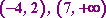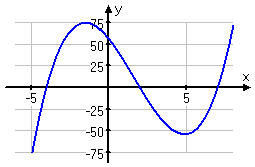As you can see, being familiar with polynomials and their shapes can make your life simpler for some of these problems. But what if you haven't learned about their shapes, or if the polynomial is more complicated, or if you have to "show your reasoning"? Your book probably prattled on about "test points", but that method requires a lot of computation, so I prefer an easier method. I'll re-work the above exercise using the "factor method".

• Find the solution: (x + 4)(x  2)(x  7) > 0
• The factors give me the zeroes of the polynomial, and the zeroes give me the following intervals of positivity and negativity: (infinity, 4), (4, 2), (2, 7), and (7, +infinity). I just need to figure out which invervals are positive and which are negative. I've got three factors, so I'll draw up a table of factors with the intervals marked off: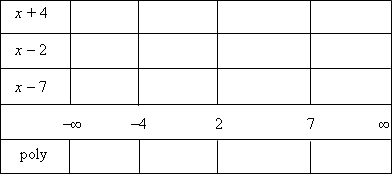This table has a row for each of the factors, a row for the number line, and a row for the polynomial. Each row is divided into columns, with each column corresponding to an interval between zeroes on the number line.

Now I'll figure out where each factor is positive. (Each factor will be negative wherever it isn't positive.)

x + 4 > 0 for x > 4
x  2 > 0 for x > 2
x  7 > 0 for x > 7

I'll mark in the table the intervals where each of the factors is positive: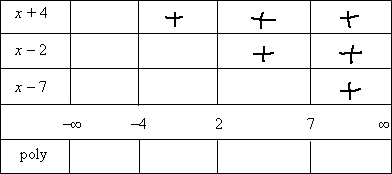...and then I'm mark the factors as being negative everywhere else: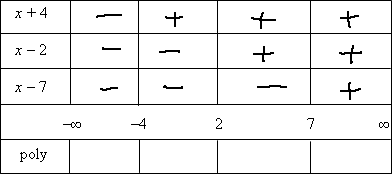The factors multiply together to create the polynomial; the signs of the factors multiply together to give the sign of the polynomial. So I'll multiply the signs of the factors on each interval to find the overall sign of the polynomial on that interval: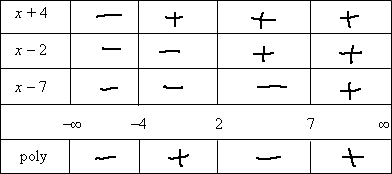In the first interval, from negative infinity to 4, there were three "minus" signs, and the product of three negatives is a negative. In the second interval, from 4 to 2, there were two "minus" signs, and the product of two negatives is a positive. And so forth.

Now I can read the solution off the table. I need the intervals where the polynomial is positive, so I'll pick the intervals that have a "plus" sign in them on the bottom row of my table:The easiest solution method for polynomial inequalities is to use what you know about polynomial shapes, but the shape isn't always enough to give you the answer. The test-point method from your book will give you the answer eventually, but it can be a lot of work. The factor-table method is quicker than test points and, since no computations are involved, it's less prone to error. So unless your instructor insists that you use the test-point method, try to learn the factor-table method. It'll make your life a lot easier.